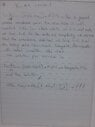# Neuronal Dynamics Passive membrane equation

• MHB
• winterdrops

#### winterdrops

Hi guys,

I am able to solve dif equation of passive membrane model. But later, author says following instructions to integrate first model. Even though I can solve the dif equation of model, I couldn't achieve the solution.

Could you help me pls. Best regards.

#### Attachments

•Int1.jpg
882.9 KB · Views: 79
Let $v = u - u_{\text{rest}}-RI_0$. Since $I(t) = I_0$, the equation becomes $T_m\, dv/dt = -v$, or $dv/v = -dt/T_m$. Integrating both sides, we obtain $\ln|v| = -t/T_m + C$ where $C$ is a constant. Thus $v = Ae^{-t/T_m}$ where $A$ is a constant. Since $v = -RI_0$ when $t = 0$, then $A = -RI_0$. Hence $v = -RI_0 e^{-t/T_m}$, or, $$u = u_{\text{rest}} + RI_0 - RI_0 e^{-t/T_m} = u_{\text{rest}} + RI_0(1 - e^{-t/T_m})$$